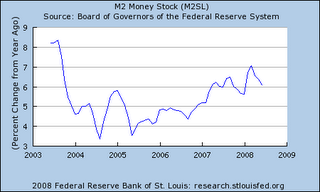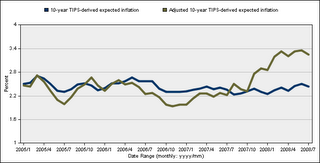## Happy birthday, Professor

Milton Friedman would have been 96 today were he still alive. Fifty events are happening around the country today to celebrate his legacy.

I have been teaching intermediate macroeconomics this summer, using the textbook of our current central bank chair (along with Andrew Abel and Dean Croushore), and we just finished the chapter on money. Towards the end of that chapter we teach the relationship between inflation and growth of the money supply. There's an equation which I can try to recreate here in words:

inflation = growth in money supply - (income elasticity of money)x(growth of real GDP)

which assumes relatively stable velocity of money in the short run. Friedman had insisted that "inflation is everywhere and always a monetary phenomenon", and I suggested we use that equation to see where we are now. I tend not to make exact measurements in class, so if I do a little rounding here you can hopefully make adjustments if you want something a little more precise.

Today's GDP report for Q2 puts the growth of GDP at about 2%. Friedman had folllowed the quantity equation to make the income elasticity of money equal one. Other research puts the number closer to 2/3 or 0.7 (the Baumol-Tobin approach makes it the inverse of the square root of 2, which is roughly 0.71). People tend to economize on cash holdings as they become wealthier, so a result for that number less than 1 makes sense.

For money, I used M2 in class (you can look at other rates here or get the raw data and do your own; see also Mark Thoma for a primer on different velocities of money). The average growth rate appears to be around 6% for the recent data.If you accept those data, then, what would you get? Assume the income elasticity is 2/3 and the growth of GDP is 2%, and your answer would be 4.33% inflation. Assume income elasticity is 1 and you get 4%.

Now, 2% isn't probably the best guess for what GDP growth will be in the long run. That might be closer to 3%. But the point is that the acceleration of money growth since early 2007 -- approximately at the beginning of the current mortgage mess, has led to easier money which Friedman would have most certainly called out as becoming inflationary at some point. The data from the TIPS market would have shown this as well.If you use 2.7% for the long run growth rate of potential GDP as CBO estimates, an expected inflation rate for the long term of 3.4% is quite reasonable for an assumption that M2 money growth stays at current levels for some time into the future. That target inflation rate of 2% is increasingly looking like it's out the window. The Fed's gift to Friedman on this birthday should be a Fed funds rate increase at its next meeting.

UPDATE: Ironman has built a little program that lets you compute your own expected inflation rate. Thanks -- very cool!

Labels: ,